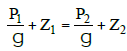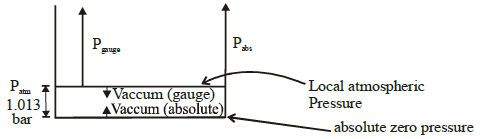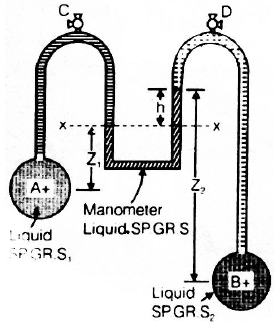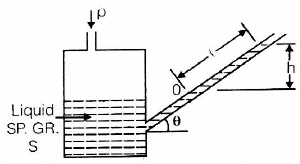Courses

# Fluid Statics Civil Engineering (CE) Notes | EduRev

## Civil Engineering (CE) : Fluid Statics Civil Engineering (CE) Notes | EduRev

The document Fluid Statics Civil Engineering (CE) Notes | EduRev is a part of the Civil Engineering (CE) Course Civil Engineering SSC JE (Technical).
All you need of Civil Engineering (CE) at this link: Civil Engineering (CE)

Chapter 2

Fluid Statics

Pascal's Law

• The intensity of pressure at any point in a stationary fluid is same in all directions.
px = py = pz

Note that pressure varies only with depth in stationary fluids, whereas if fluids is in motion, pressure may vary in horizontal directions also.

• Fluid pressure is measured in Force/ Area and it is expressed in Pascal (N/m 2) or Bar. 1 Bar = 105 N/m2 1 MPa = 10 Bar. For static fluids, piezo-metric head is constant throughout the fluid.Pressure Scales (i) Absolute Scale

• Pressure is measured with reference to absolute zero. In this scale pressure cannot be negative. Minimum pressure is zero.
• Abs. pressure = Pguage + local atm. pressure

(ii) Gauge Scale: Pressure is measured with reference to atmospheric pressure. It means atmospheric pressure is taken zero. Gauge pressure can be positive, negative or zero.

• Gauge pressure = Absolute pressure – local atmospheric pressure
• Vacuum pressure = local atmospheric pressure – absolute pressure
• The atmospheric pressure varies with altitude, temperature and local conditions. It is measured by Barometer.
• At mean sea level atmospheric pressure is 1.01 × 105 Pascal or 1 Bar or 10.3 mts. of height of water or 76 cm height of mercury.

Pressure Measuring Devices:

(i) Piezometer: It is simple graduated glass tube which measures pressure in gauge scale. The height up to which liquid is raised in this tube is called pressure head. It is useful for small pressure measurement and is not suitable for high pressure and negative pressure measurements. p = gh; where 'h' is height of liquid column

(ii) Manometer It is based on liquid column balance mechanism. The manometric liquid used should have high density & low vapour pressure.

(a) Simple Manometer or U-tube Manometer.
It can measure both positive and negative pressures.
(b) Differential Manometer.
It measures difference in pressure between two points.(c) Micromanometer : It measures small difference of pressure. It is modified form of Simple Manometer whose one  limb is made of larger cross section area.

(d) Inclined Manometer : It measures small pressure low velocity gas flow.p = S gw
sin q =gℓ sinq
S = specific gravity of liquid
gw = specific weight of water
g = specific weight of manometric liquid

(iii) Mechanical Gauges: These are used for rough measurement of high pressure. These are based on spring balance mechanism. Ex. Bourdon tube pressure gauge.

(iv) Aneroid Barometer or Mercury Barometer: It is used to measure local atmospheric pressure on absolute scale.

Offer running on EduRev: Apply code STAYHOME200 to get INR 200 off on our premium plan EduRev Infinity!

## Civil Engineering SSC JE (Technical)

109 docs|50 tests

,

,

,

,

,

,

,

,

,

,

,

,

,

,

,

,

,

,

,

,

,

;